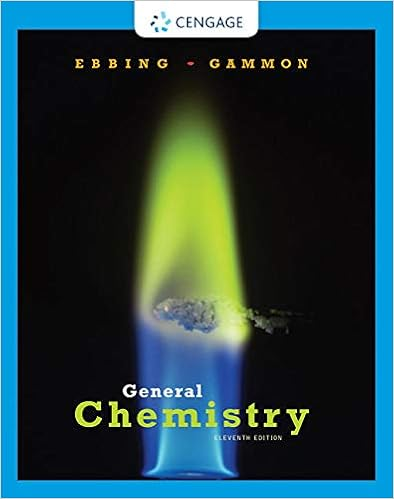# equiexcelac05 - Chemistry Excel Equilibrium Activity Name...

• Notes
• UltraLightningCobra8457
• 2

This preview shows page 1 - 2 out of 2 pages.

##### We have textbook solutions for you!
The document you are viewing contains questions related to this textbook.The document you are viewing contains questions related to this textbook.
Chapter 18 / Exercise 18.80
General Chemistry
Ebbing/GammonExpert Verified
Chemistry Excel Equilibrium Activity Name _________________________________________ Date ______________ Period _____ equiexcelac05.doc Show all calculations. Introduction: Given the following chemical equation: 3H2(g)+ CO(g)H2O(g)+ CH4(g)Kc= 190 @ 1000K written in the form aA + bB cC + dD You will use an Excel spreadsheet to investigate and determine equilibrium conditions given a set of initial concentrations. Open the appropriate Excel spreadsheet (excelequilact2005.xls). Familiarize yourself with the layout of the application. For the moment, focus on the boxed area in dark blue. The boxes in this area are where you will input coefficients, initial concentrations, Kcvalues and make manual changes to the concentrations. This area also keeps you informed about the current Q (mass-action expression) value for the reaction. The light purple area will show the current concentrations for each chemical component. Procedure: 1. Examine the Kcvalue given above. Does this reaction appear to be spontaneous? Explain. 2.
##### We have textbook solutions for you!
The document you are viewing contains questions related to this textbook.The document you are viewing contains questions related to this textbook.
Chapter 18 / Exercise 18.80
General Chemistry
Ebbing/GammonExpert Verified
•••